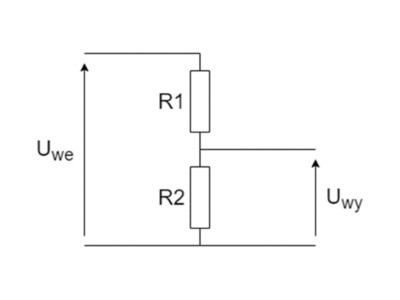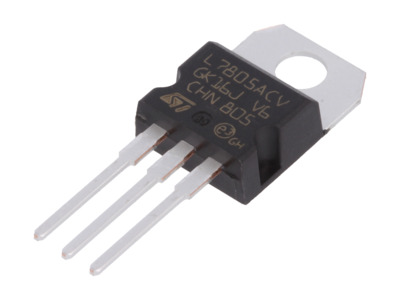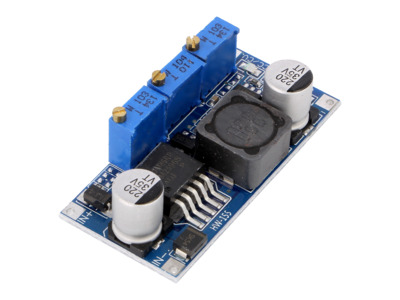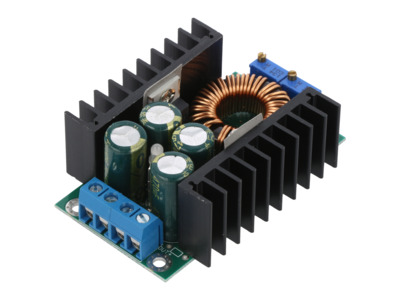# DC voltage converter — how to choose the right one?

Transfer Multisort Elektronik

Monday, 11 July, 2022

Voltage converter, voltage divider, linear stabiliser — which one should you choose?

Every electronic device requires a suitable power source. In most cases we simply insert the plug into the socket. It is worth noting, however, that there are very few devices powered by variable voltage of 230 V, which is available from the socket, while much more commonly it is constant voltage of much lower electric potential that is required. To supply such voltage, manufacturers design appropriate, often very extensive, power supply sections, or equip their devices with a port to which you can plug the connector of a standard plug-in power supply. It may also happen that the correct operation of the device requires several voltages with different values. In this case, the developers have to appropriately lower or sometimes increase the input voltage.

#### Three power supply methods: voltage converter, voltage divider, linear stabiliser

There are basically three ways to supply power: the power supply section can be based on a voltage divider, a linear stabiliser or a voltage converter. Each of these methods can be applied to lower the voltage, but only converters allow to increase the voltage.

##### Voltage dividers

The first method of implementing the power supply section that you may encounter is based on a voltage divider. The operation of such a circuit is based on Ohm’s law and the voltage deposited on individual resistors. By manipulating their value, you can get a voltage of any value at the output, but it will be lower than the input voltage. However, this way of powering the device has some disadvantages. The first of them is the current efficiency — a voltage divider is made of resistors whose power is usually very low. Any higher current will cause the resistors to burn very quickly. Of course, you can use more powerful resistors, but this would considerably increase the price of such a circuit. Another major disadvantage is that the divider is always designed with a specific input voltage in mind. If it is increased or decreased, the output voltage will also change.Figure 1: A diagram of a simple voltage divider.

##### Linear stabilisers

Linear stabilisers are another solution to lower the input voltage. Produced mostly in the form of components with three legs, they are quite common in consumer electronics. Their task is very simple — to maintain a constant value of voltage at the output, regardless of the input voltage. Of course, it should be remembered that the input voltage must be higher than the output voltage. Components of this type are characterised by a low price, but have a problem of heat dissipation. Any linear stabiliser must convert the difference between the input voltage and the desired output voltage into heat energy. This results in the fact that even at low currents, the component heats up very quickly, due to which additional heat sinks must be used, which of course translates into higher costs.Figure 2: Linear stabiliser L7805ACV.

##### Voltage converters

A third possibility of implementing a power supply system is to base it on a voltage converter. It consists of several basic components: a coil, a switching transistor, capacitors, resistors and a silicon diode. A voltage converter is a much more complex device compared to the previously presented solutions. They come in several variants and can both increase and decrease the output voltage in relation to the input voltage.Figure 3: Voltage converter OKY3497-5.

#### Why is it worth choosing a voltage converter?

Voltage converters are characterised by one feature that is useful in the process of designing electronic devices, namely efficiency. As you already know, power losses are quite a big disadvantage of linear stabilisers. In this respect, converters are much more economical. Every voltage converter has a parameter defined as efficiency, which is expressed as a percentage and refers to the ratio of the output power to the power drawn from the source. In other words, if the device has an efficiency level of 80%, it means that 20% of power is lost in the form of heat dissipated mainly by the transistor and the coil. However, it is important to know that the efficiency is not a constant value and depends on the operating conditions of the converter, mainly on the supply voltage, output voltage and load current.

Beside their advantages, voltage converters also have a disadvantage, ie, interference. Due to their design and operating principle, the output voltage of an inverter is not perfectly constant. This can be a problem if we need to power a circuit that needs a perfectly constant voltage. In such a case it is necessary to place an RC filter at the output of the converter.

Thanks to their compact dimensions and high efficiency, voltage converters can be used virtually anywhere. When designing any device, it is worth choosing this type of component.

#### Voltage converter — how does it work?

A voltage converter is a pulse element that, in simple terms, reduces voltage by switching it (alternating on and off). This process is so fast that it does not interfere with the operation of the powered device or system. The element controlling the switching in DC/DC converters is usually a MOSFET. However, for the DC voltage to appear at the output of the device, additional elements such as capacitors and an inductor (choke) are needed. While capacitors only play the role of filters here, the inductive element has the task of storing energy while the device is working, in order to return it later. In addition, a silicon diode controlling the direction of the flowing current must be placed in the converter circuit.

#### Voltage converter: types

There are several types of voltage converters available on the market, the most popular being:

• step-down (buck) converter — reduces voltage
• step-up (boost) converter — increases voltage
• step-up/down (SEPIC) converter — reduces or increases the output voltage.Figure 4: Voltage converter OKY3497-4.

In addition, there are other designs, the most interesting of which include:

• Forward converter — a type of DC/DC converter based on a transformer. It is a single-switch element — the energy drawn from the source is transferred to the output in real time. Thanks to the use of a transformer, the forward converter allows the input and output to be galvanically separated. Forward converters are used in switching power supplies with a power output of less than a few hundred watts and in inverter welders.
• Flyback converter — a type of DC/DC converter which also uses galvanic separation of input and output. It is a two-switch device; the energy is accumulated in the magnetic field of the coil in the first phase and returned in the second phase. Systems of this type are used primarily in low-power switched-mode power supplies.

#### Voltage converter: what should you consider when choosing one?

The choice of a suitable converter depends mainly on what device you are designing. If you want to design a small portable device, it is worth considering miniature converters. For larger equipment, you can use converters of bigger dimensions with a built-in heat sink to facilitate heat dissipation. Additionally, it is necessary to specify the type of converter needed — step-up, step-down or step-up/down.

If you wish to minimise energy losses, it is worth equipping yourself with voltage converters of the highest possible efficiency. The standard efficiency of such devices falls within the range of 80–95%. Additionally, it is worth remembering about the basic parameters of the converter, such as: maximum output current, output voltage range and input voltage range.

Originally published here.

Related Articles

#### Engineers solve a mystery on the path to smaller, lighter batteries

Branchlike metallic filaments can sap the power of solid-state lithium batteries. A new study...

#### Metal-free batteries raise hope for more sustainable and economical grids

Ammonium-ion electrolytes could help create ecofriendly and sustainable alternatives to...

#### High energy X-rays pave the way for better EV batteries

Scientists have used high energy X-rays to study the solid-electrolyte interphase, a chemical...